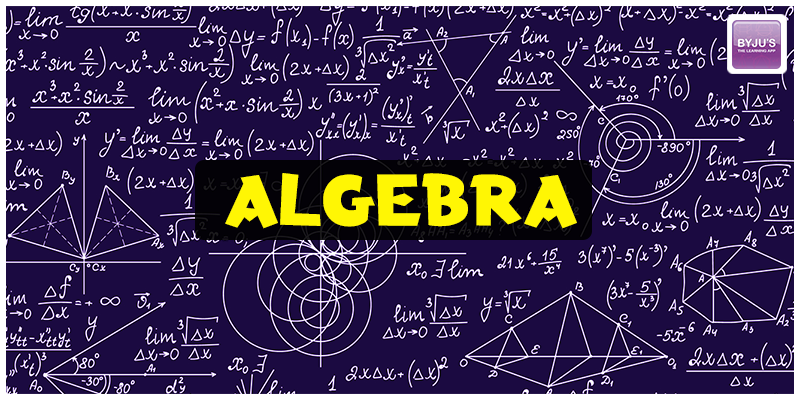# Important Basic Algebra Formulas For GMAT

##Staring at the GMAT quant section might look daunting at first as most of the syllabus is from your higher secondary education. If you had paid full attention to the teacher while they were writing down the formulas on the blackboard, then you might not be feeling that scared at all. But you need not to worry, use this page as a cheat sheet for all the comprehensive formulas based questions that might rock the GMAT ship.

This GMAT Quant cheat sheet contains all the formulas necessary to crack the section, right from Arithmetic, Algebra, and Geometry, which are part of Problem Solving and Data Sufficiency.

## GMAT Quantitative: Problem Solving

The problem-solving segment in the Quantitative section of the GMAT is designed to test the candidate’s ability in solving math problems using various mathematical formulas. The three main chapters from which questions are asked are Arithmetic, Algebra and Geometry.

## Arithmetic Formulas

General Formulas:

$a^{2} – b^{2} = (a+b)\times (a-b)$ $(a + b)^{2} = a^{2} + 2ab + b^{2} = (a-b)^{2} + 4ab$ $(a – b)^{2} = a^{2} – 2ab + b^{2} = (a+b)^{2} – 4ab$ $a^{2} + b^{2} = (a+b)^{2} -2ab = (a-b)^{2} + 2ab$ $a^{3} + b^{3} = (a+b)(a^{2} -ab +b^{2}) = (a+b)^{3} – 3ab(a+b)$ $a^{3} – b^{3} = (a – b)(a^{2} + ab + b^{2}) = (a – b)^{3} – 3ab(a-b)$ $(a + b)^{3} = a^{3} + 3a^{2}b + 3ab^{2} + b^{3} = a^{3} + b^{3}+ 3ab(a + b)$ $(a – b)^{3} = a^{3} – 3a^{2}b + 3ab^{2} – b^{3} = a^{3} – b^{3} – 3ab(a – b)$ $(x + a)(x + b) = x^{2} + x(a + b) + ab$ $(x – a)(x + b) = x^{2} + x(b – a) – ab$ $(x – a)(x – b) = x^{2} – x(a + b) + ab$ $(a + b + c)^{2} = a^{2} + b^{2} + c^{2} + 2ab + 2bc + 2ac$ $(a – b – c)^{2} = a^{2} + b^{2} + c^{2} – 2ab + 2bc – 2ac$ $(a + b + c)^{3} = a^{3} + b^{3} + c^{3} + 3(a + b)(b + c)(c + a)$

## Laws of Exponents

Multiplying two exponents with same base, then the result is the base to the power of sum of the powers.

$x^{m} \times x^{n} = x^{(m+n)}$ $x^{m} \times x^{n} … \times x^{p} = x^{(m+n…+p)}$

Dividing two exponents with the same base, then the result will be the base to the power of difference of the powers.

$\frac{x^{m}}{x^{n}} = x^{m}\div x^{n} = x^{(m – n)}$ $(x^{m})^{n} = x^{(mn)}$ $x^{0} = 1$ $x^{-m} = \frac{1}{x^{m}}$ $x^{m} = \frac{1}{x^{-m}}$ $x^{\frac{m}{n}} = \sqrt[n]{x^{m}}$ $\left ( \frac{x^{a}}{y^{b}} \right )^{c} = \frac{x^{ac}}{y^{bc}}$ $\frac{x^{m}}{y^{m}} = \left ( \frac{x}{y} \right )^{m}$ $x^{\frac{p}{q}} = \sqrt[q]{x^{p}} = (\sqrt[q]{x})^{p}$ $\sqrt[m]{\frac{x}{y}} = \frac{\sqrt[m]{x}}{\sqrt[m]{y}}$ $(-1)^{n} = \left\{\begin{matrix} 1 & , n \; even\\ -1 & n \; odd \end{matrix}\right.$

$a^{\frac{x}{y}} = \sqrt[y]{a^{x}}$ $\sqrt{x}.\sqrt{n} = \sqrt{xn}$ $\sqrt{\frac{x}{y}} = \frac{\sqrt{x}}{\sqrt{y}}$ $(\sqrt{x})^{n} = \sqrt{x^{n}}$ $a\sqrt{c} + b\sqrt{c} = (a+b)\sqrt{c})$ $\sqrt{a} + \sqrt{b} \neq \sqrt{a+b}$

## Number Properties

(Odd) * (Even) = Even

(Odd) * (Odd) = Odd

(Even) * (Even) = Even

(Odd) ± (Even) = Odd

(Odd) ± (Odd) = Even

(Even) ± (Even) = Even

(Positive) * (Positive) = Positive

(Positive) * (Negative) = Negative

(Negative) * (Negative) = Positive

(Positive)/(Negative) = Negative

(Positive)/(Positive) = Positive

(Negative)/(Negative) = Positive

### GMAT Algebra Formulas

$ax^{2} + bx + c = 0$

$x=\frac{-b\pm \sqrt{b^{2}-4ac}}{2a}$

$a^{2} – b^{2} = (a+b)(a-b)$

$a^{2} + 2ab + b^{2} = (a+b)^{2}$

$a^{2} – 2ab + b^{2} = (a-b)^{2}$

Interest

$Simple \; Interest = \frac{Prt}{100}$

Where,

P = starting principle
r = annual interest rate
t = number of years

### Area & Perimeter formulas

Square: Area: (length)2 | Perimeter: 4(length)

Parallelogram: Area: base X height | Perimeter: 2(base) + 2(height)

Circle: Area: πr2 | Circumference of a circle: 2πr [where Pi (π) = 3.14]

Triangle: Area: (1/2) length X breadth

Pythagoras Theorem (for right angled triangles): (base)2 + (height)2 = (hypotenuse)2

### Volume formulas

Cube: (length)3

Rectangular prism: length X breadth X height

Cylinder: πr2h

Cone: (1/3) πr2h

Pyramid: (1/3) base length X base width X height

Sphere: (4/3)πr3

## GMAT Quantitative: Data Sufficiency

This segment of the GMAT Quantitative section tests the ability of the candidate’s to solve questions through the given data. These questions require you to use the logical, analytical and quantitative skills to answer the questions.

## Statistics

Mean = $A = \frac{S}{N}$

Where,

A = average (or arithmetic mean)

N = the number of terms (e.g., the number of items or numbers being averaged)

S = the sum of the numbers in the set of interest (e.g., the sum of the numbers being averaged)

Standard Deviation = $\sigma = \sqrt{\frac{\sum_{i=1}^{n}(x_{i}-\mu )^{2}}{n}}$

$\sigma$ = Standard Deviation

$\mu$ = Mean

n = Number of elements in Set

### Permutation and Combination

Permutation formula: nPr = n! / (n-r)!

Combination formula: nCr = n! / (r!)(n-r)!

### Formulas for Word Problems

Probability = Number of favourable outcomes / Number of all possible outcomes

Probability of events A & B happening = Probability of A X Probability of B

Probability of either event A or B happening = Probability of A + Probability of B

Read more GMAT Quant related articles.

BYJU’S will be glad to help you in your GMAT preparation journey. You can ask for any assistance related to GMAT and MBA from us by calling us at +918884544444. You can write to us at gmat@byjus.com.